# Numeral Systems Part II Subjects Hexadecimal Converting BinarytoHexadecimal

• Slides: 40Numeral Systems (Part II) Subjects: -Hexadecimal -Converting Binary-to-Hexadecimal or Hexadecimal-to-Binary -Converting Binary-to-Octal or Octal-to-Binary -Computer Character Sets and Data RepresentationThe Hexadecimal Number System The hexadecimal (base 16) number system is a positional number system as are the decimal number system and the binary number system. Recall that in any positional number system, regardless of the base, the highest numerical symbol always has a value of one less than the base. Furthermore, one and only one symbol must ever be used to represent a value in any position of the number. For number systems with a base of 10 or less, a combination of Arabic numerals can be used to represent any value in that number system. The decimal number system uses the Arabic numerals 0 through 9; the binary number system uses the Arabic numerals 0 and 1; the octal number system uses the Arabic numerals 0 through 7; and any other number system with a base less than 10 would use the Arabic numerals from 0 to one less than the base of that number system. However, if the base of the number system is greater than 10, more than 10 symbols are needed to represent all of the possible positional values in that number system. ! The hexadecimal number system uses not only the Arabic numerals 0 through 9, but also uses the letters A, B, C, D, E, and F to represent the equivalent of 1010 through 1510, respectively.The Hexadecimal Number System For reference, the following table shows the decimal numbers 0 through 31 with their hexadecimal equivalents: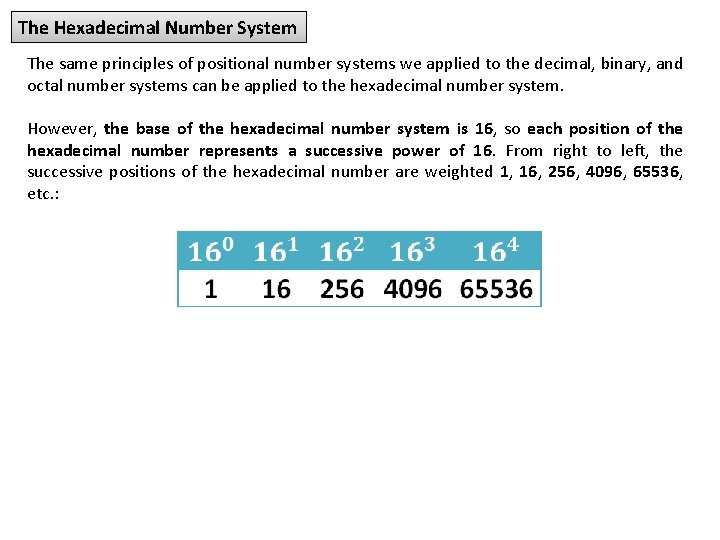The Hexadecimal Number System The same principles of positional number systems we applied to the decimal, binary, and octal number systems can be applied to the hexadecimal number system. However, the base of the hexadecimal number system is 16, so each position of the hexadecimal number represents a successive power of 16. From right to left, the successive positions of the hexadecimal number are weighted 1, 16, 256, 4096, 65536, etc. :Converting a Hexadecimal Number to a Decimal Number We can use the same method that we used to convert binary numbers and octal numbers to decimal numbers to convert a hexadecimal number to a decimal number, keeping in mind that we are now dealing with base 16. From right to left, we multiply each digit of the hexadecimal number by the value of 16 raised to successive powers, starting with the zero power, then sum the results of the multiplications. Remember that if one of the digits of the hexadecimal number happens to be a letter A through F, then the corresponding value of 10 through 15 must be used in the multiplication. Example 1: Convert the hexadecimal number 20 B 316 to its decimal equivalent.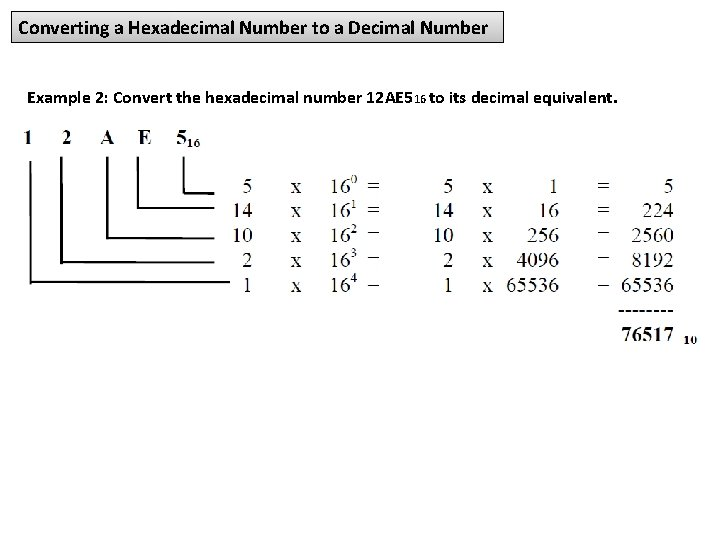Converting a Hexadecimal Number to a Decimal Number Example 2: Convert the hexadecimal number 12 AE 516 to its decimal equivalent.Converting a Decimal Number to a Hexadecimal Number To convert a decimal number to its hexadecimal equivalent, the remainder method (the same method used in converting a decimal number to its binary equivalent) can be used. To review, the remainder method involves the following four steps: ! (1) Divide the decimal number by the base (in the case of hexadecimal, divide by 16). (2) Indicate the remainder to the right. If the remainder is between 10 and 15, indicate the corresponding hex digit A through F. (3) Continue dividing into each quotient (and indicating the remainder) until the divide operation produces a zero quotient. (4) The base 16 number is the numeric remainder reading from the last division to the first (if you start at the bottom, the answer will read from top to bottom).Converting a Decimal Number to a Hexadecimal Number Example: Convert 926310 to its hexadecimal equivalent: 1) Divide 16 into 9263. The quotient is 578 with a remainder of 15; so indicate the hex equivalent, "F", on the right. 2) Divide 16 into 578 (the quotient from the previous division). The quotient is 36 with a remainder of 2, indicated on the right. 3) Divide 16 into 36. The quotient is 2 with a remainder of 4, indicated on the right. 4) Divide 16 into 2. The quotient is 0 with a remainder of 2, as indicated. Since the quotient is 0, stop here. The answer is 242 F, so 926310 = 242 F 16.Hexadecimal Addition One consideration is that if the result of an addition is between 10 and 15, the corresponding letter A through F must be written in the result. In the example 5 + 9 = 14, so an "E" was written in that position; 9 + 1 = 10, so an "A" was written in that position. A second consideration is that if either of the addends contains a letter A through F, convert the letter to its decimal equivalent (either by memory or by writing it down) and then proceed with the addition. A third consideration is that if the result of an addition is greater than 15, you must subtract 16 from the result of that addition, put down the difference of that subtraction for that position, and carry a 1 over to the next position. In the example when B 16 (1110) was added to E 16 (1410), the result was 2510. Since 2510 is greater than 1510, we subtracted 1610 from the 2510 to get 910. We put the 9 down and carried the 1 over to the next position.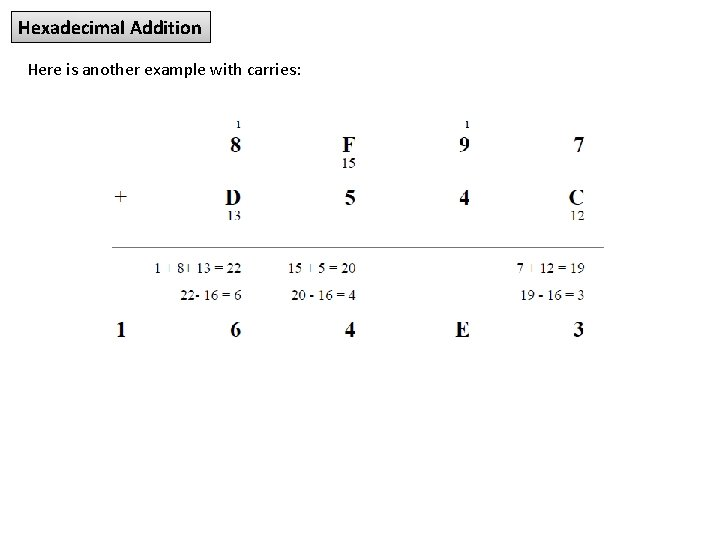Hexadecimal Addition Here is another example with carries:Hexadecimal Subtraction We will use the complement method to perform hexadecimal subtraction. The steps for subtracting two hexadecimal numbers are as follows: ! (1) Compute the 15's complement of the subtrahend by subtracting each digit of the subtrahend by 15. (2) Add 1 to the 15's complement of the subtrahend to get the 16's complement of the subtrahend. (3) Add the 16's complement of the subtrahend to the minuend and drop the high-order 1. This is your difference. Example: Compute ABED 16 – 1 FAD 16 (1) Compute the 15's complement of 1 FAD 16 by subtracting each digit from 15: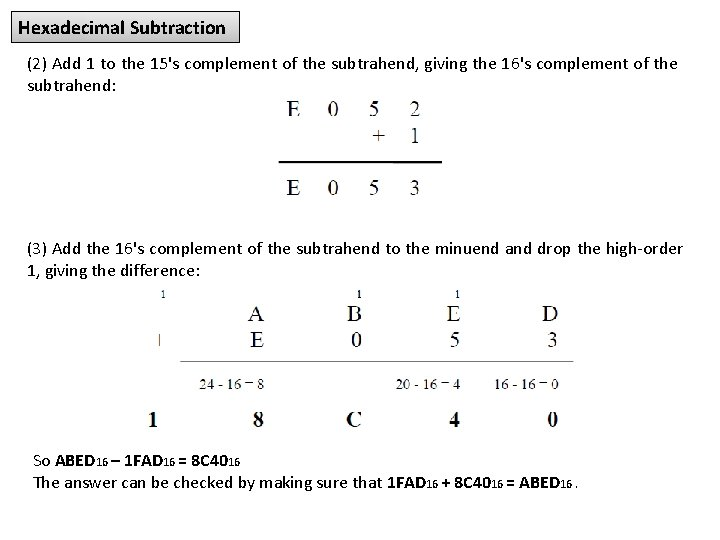Hexadecimal Subtraction (2) Add 1 to the 15's complement of the subtrahend, giving the 16's complement of the subtrahend: (3) Add the 16's complement of the subtrahend to the minuend and drop the high-order 1, giving the difference: So ABED 16 – 1 FAD 16 = 8 C 4016 The answer can be checked by making sure that 1 FAD 16 + 8 C 4016 = ABED 16.Converting Binary-to-Hexadecimal or Hexadecimal-to-Binary Converting a binary number to its hexadecimal equivalent or vice-versa is a simple matter. Four binary digits are equivalent to one hexadecimal digit, digit as shown in the table below:Converting Binary-to-Hexadecimal or Hexadecimal-to-Binary To convert from binary to hexadecimal, divide the binary number into groups of 4 digits starting on the right of the binary number. If the leftmost group has less than 4 bits, put in the necessary number of leading zeroes on the left. For each group of four bits, write the corresponding single hex digit. To convert from hexadecimal to binary, write the corresponding group of four binary digits for each hex digit.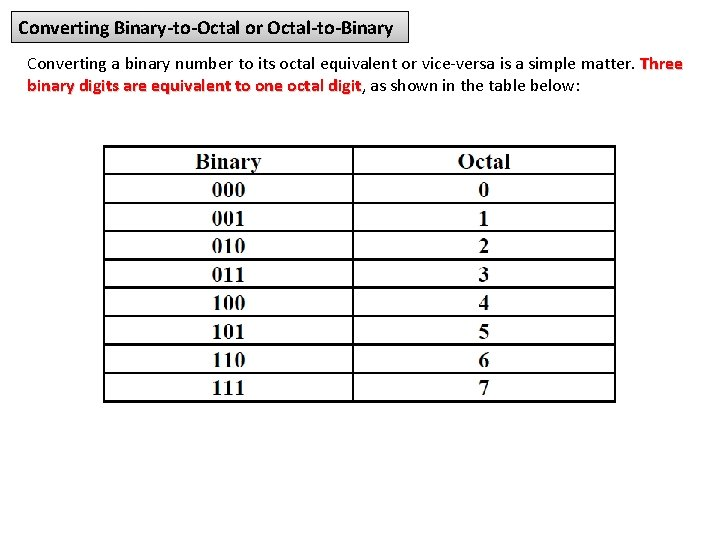Converting Binary-to-Octal or Octal-to-Binary Converting a binary number to its octal equivalent or vice-versa is a simple matter. Three binary digits are equivalent to one octal digit, digit as shown in the table below: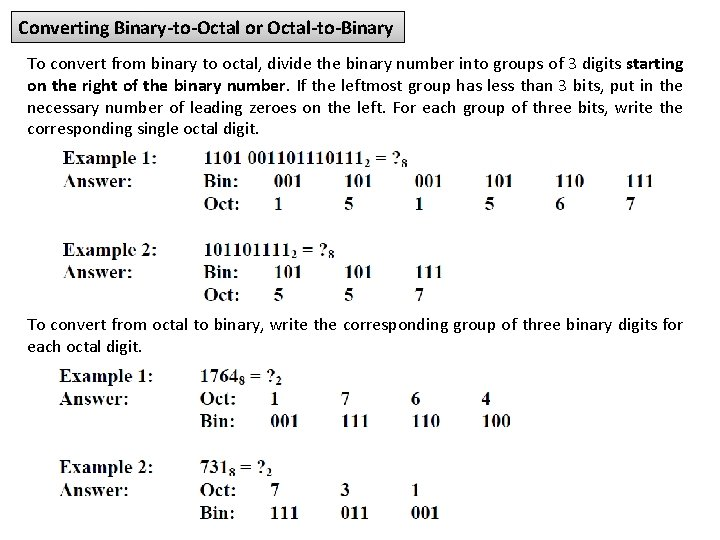Converting Binary-to-Octal or Octal-to-Binary To convert from binary to octal, divide the binary number into groups of 3 digits starting on the right of the binary number. If the leftmost group has less than 3 bits, put in the necessary number of leading zeroes on the left. For each group of three bits, write the corresponding single octal digit. To convert from octal to binary, write the corresponding group of three binary digits for each octal digit.Data Representation Data Formats Computers process and store all forms of data in binary format. Human communication includes language, images and sounds. Data formats are specifications for converting data into computer-usable form. Lets define the different ways human data may be represented, stored and processed by a computer. Computing Systems Data Computing systems are complex devices, dealing with a vast array of information categories. The computing systems store, present, and help us modify: ü ü Text; Audio; Images and graphics; Video.Data Representation Computing systems are finite machines. They store a limited amount of information, even if the limit is very big. The goal is to represent enough of the real world data to satisfy our computational needs and our senses of sight and sound. The information can be represented in one of two ways: analog or digital Analog data is a continuous representation, analogous to the actual information it represents. Digital data is a discrete representation, breaking the information up into separate (discrete) elements. Computers cannot work with analog information directly, so there is a need to digitize the analog information. This is done by breaking the analog information into pieces and representing those pieces using binary digitsData Representation Both electronic signals (analog and digital) degrade as they move down a line. The voltage of the signal fluctuates due to environmental effects. As soon as an analog signal degrades, information is lost. Since any voltage level within the range is valid, it is impossible to know that the original signal was even changed. Digital signals jump sharply between two extremes (high and low state). A digital signal can degrade quite a bit until the information is lost, because any value over a certain threshold is considered high value and below the threshold is considered low value. You can still retrieve the information from a reasonably degraded digital signal. Periodically a digital signal is reclocked to regain its original shape. As long as it is reclocked before too much degradation, no information is lost.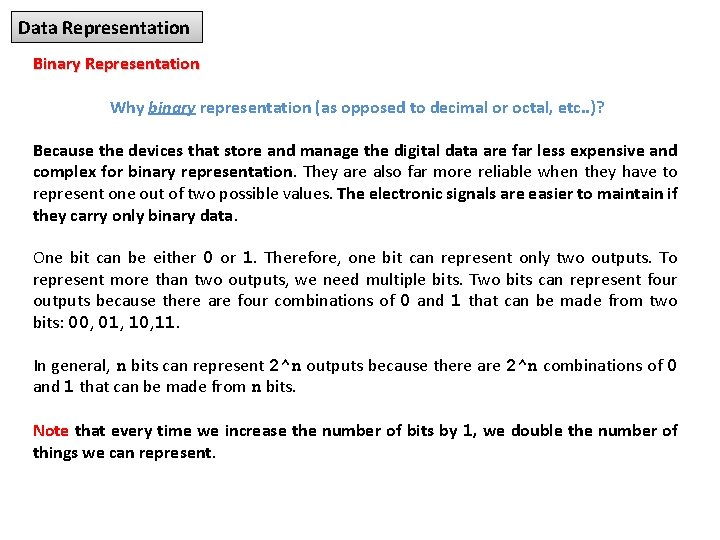Data Representation Binary Representation Why binary representation (as opposed to decimal or octal, etc. . )? Because the devices that store and manage the digital data are far less expensive and complex for binary representation. They are also far more reliable when they have to represent one out of two possible values. The electronic signals are easier to maintain if they carry only binary data. One bit can be either 0 or 1. Therefore, one bit can represent only two outputs. To represent more than two outputs, we need multiple bits. Two bits can represent four outputs because there are four combinations of 0 and 1 that can be made from two bits: 00, 01, 10, 11. In general, n bits can represent 2^n outputs because there are 2^n combinations of 0 and 1 that can be made from n bits. Note that every time we increase the number of bits by 1, we double the number of things we can represent.Data Formats – How to Interpret Data Meaning of internal representation must be appropriate for the type of processing to take place: q That is, images and sound have to be digitized. ü Images need detailed description of the data, how color is represented at each data point. ü Sound need sampling rate. q Proprietary formats unique to a product or company. For example, Microsoft Word, Corel Word Perfect, IBM Lotus Notes. q Standards evolve two ways: 1. Proprietary formats become de facto standards (e. g. , Adobe Post. Script, Apple Quick Time). 2. Committee is struck to solve a problem (Motion Pictures Experts Group, MPEG).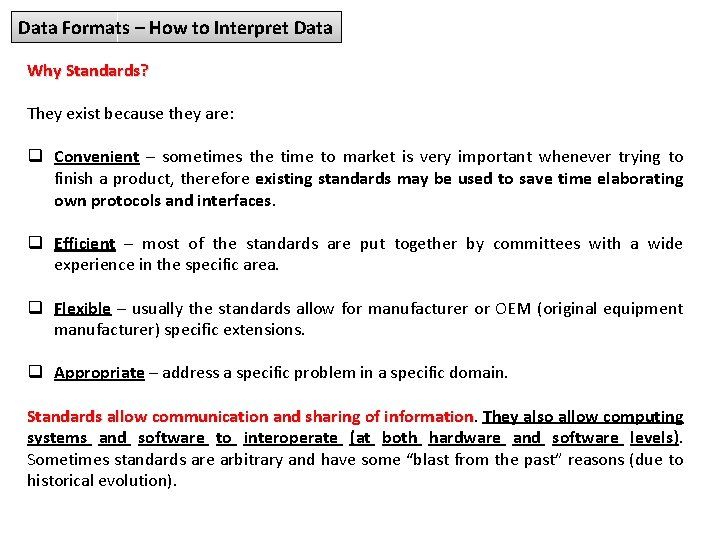Data Formats – How to Interpret Data Why Standards? They exist because they are: q Convenient – sometimes the time to market is very important whenever trying to finish a product, therefore existing standards may be used to save time elaborating own protocols and interfaces. q Efficient – most of the standards are put together by committees with a wide experience in the specific area. q Flexible – usually the standards allow for manufacturer or OEM (original equipment manufacturer) specific extensions. q Appropriate – address a specific problem in a specific domain. Standards allow communication and sharing of information They also allow computing systems and software to interoperate (at both hardware and software levels). Sometimes standards are arbitrary and have some “blast from the past” reasons (due to historical evolution).Data Formats – How to Interpret Data Examples of Standards Organizations ISO – International Standards Organization ANSI – American National Standards Institute IEEE – Institute for Electrical and Electronics Engineers Text: Alphanumeric Data. Standards for representing letters (alpha) and numbers: q ASCII – American Standard Code for Information Interchange. q EBCDIC – Extended Binary-Coded Decimal Interchange Code (not used anymore, used to be used in IBM mainframes). q Unicode.Character Encoding A character encoding system consists of a code that pairs each character from a given repertoire with something else — such as a bit pattern, sequence of natural numbers, octets, or electrical pulses — in order to facilitate the transmission of data (generally numbers or text) through telecommunication networks or for data storage. Code unit The code unit, is a unit used for character encoding. ü ü ü With US-ASCII, code unit is 7 bits. With UTF-8, code unit is 8 bits. With EBCDIC, code unit is 8 bits. With UTF-16, code unit is 16 bits. With UTF-32, code unit is 32 bits. Then the encoding attributes to single code unit or sequence of code unit a meaning.ASCII Character Encoding The name ASCII is an acronym for: American Standard Code for Information Interchange. It is a character encoding standard developed several decades ago to provide a standard way for digital machines to encode characters. The ASCII code provides a mechanism for encoding alphabetic characters, numeric digits, and punctuation marks for use in representing text and numbers written using the Roman alphabet. As originally designed, it was a seven bit code. The seven bits allow the representation of 128 unique characters. All of the alphabet, numeric digits and standard English punctuation marks are encoded. The ASCII standard was later extended to an eight bit code (which allows 256 unique code patterns) and various additional symbols were added, including characters with diacritical marks (such as accents) used in European languages, which don’t appear in English. There also numerous non-standard extensions to ASCII giving different encoding for the upper 128 character codes than the standard. ISO/IEC 8859 -1: 1998, Information technology — 8 -bit single-byte coded graphic character sets — Part 1: Latin alphabet No. 1, is part of the ISO/IEC 8859 series of ASCIIbased standard character encodings, first edition published in 1987.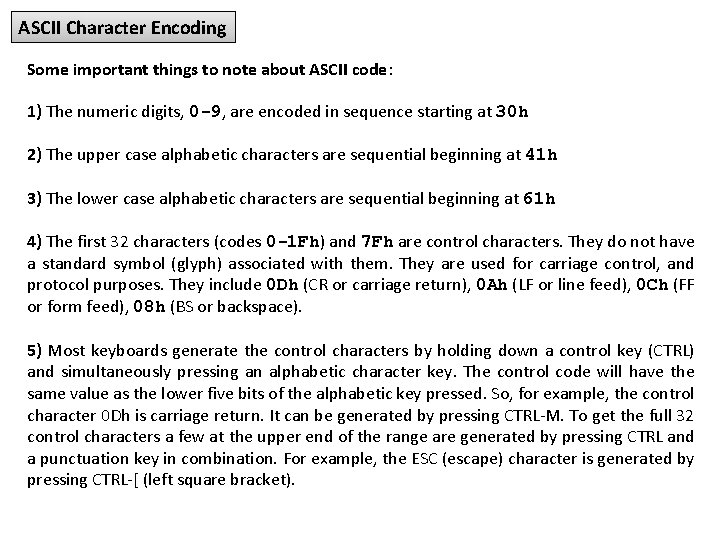ASCII Character Encoding Some important things to note about ASCII code: 1) The numeric digits, 0 -9, are encoded in sequence starting at 30 h 2) The upper case alphabetic characters are sequential beginning at 41 h 3) The lower case alphabetic characters are sequential beginning at 61 h 4) The first 32 characters (codes 0 -1 Fh) and 7 Fh are control characters. They do not have a standard symbol (glyph) associated with them. They are used for carriage control, and protocol purposes. They include 0 Dh (CR or carriage return), 0 Ah (LF or line feed), 0 Ch (FF or form feed), 08 h (BS or backspace). 5) Most keyboards generate the control characters by holding down a control key (CTRL) and simultaneously pressing an alphabetic character key. The control code will have the same value as the lower five bits of the alphabetic key pressed. So, for example, the control character 0 Dh is carriage return. It can be generated by pressing CTRL-M. To get the full 32 control characters a few at the upper end of the range are generated by pressing CTRL and a punctuation key in combination. For example, the ESC (escape) character is generated by pressing CTRL-[ (left square bracket).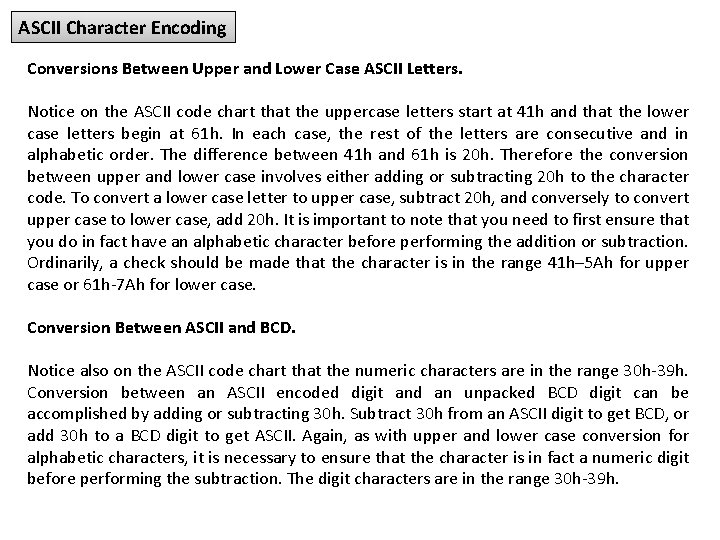ASCII Character Encoding Conversions Between Upper and Lower Case ASCII Letters. Notice on the ASCII code chart that the uppercase letters start at 41 h and that the lower case letters begin at 61 h. In each case, the rest of the letters are consecutive and in alphabetic order. The difference between 41 h and 61 h is 20 h. Therefore the conversion between upper and lower case involves either adding or subtracting 20 h to the character code. To convert a lower case letter to upper case, subtract 20 h, and conversely to convert upper case to lower case, add 20 h. It is important to note that you need to first ensure that you do in fact have an alphabetic character before performing the addition or subtraction. Ordinarily, a check should be made that the character is in the range 41 h– 5 Ah for upper case or 61 h-7 Ah for lower case. Conversion Between ASCII and BCD. Notice also on the ASCII code chart that the numeric characters are in the range 30 h-39 h. Conversion between an ASCII encoded digit and an unpacked BCD digit can be accomplished by adding or subtracting 30 h. Subtract 30 h from an ASCII digit to get BCD, or add 30 h to a BCD digit to get ASCII. Again, as with upper and lower case conversion for alphabetic characters, it is necessary to ensure that the character is in fact a numeric digit before performing the subtraction. The digit characters are in the range 30 h-39 h.ASCII Character Encoding Each character is stored in the computer as a byte. Since a byte consists of eight bits, there are 28, or 256 possible combinations of bits within a byte, numbered from 0 to 255. ASCII Character Set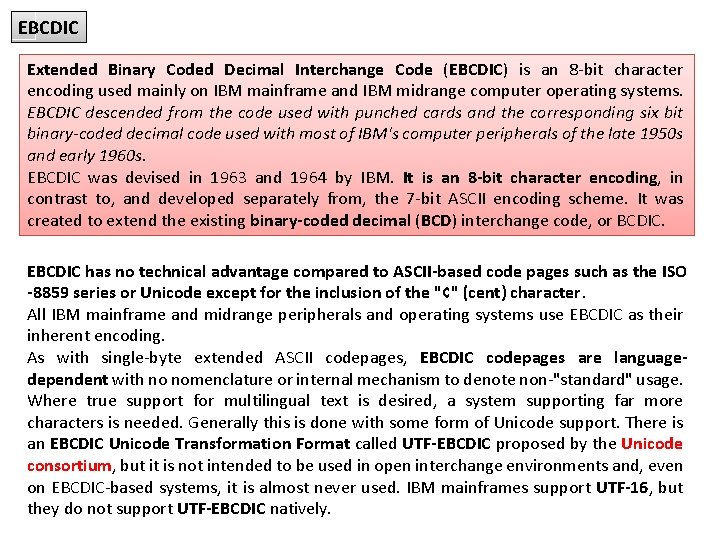EBCDIC Extended Binary Coded Decimal Interchange Code (EBCDIC) is an 8 -bit character encoding used mainly on IBM mainframe and IBM midrange computer operating systems. EBCDIC descended from the code used with punched cards and the corresponding six bit binary-coded decimal code used with most of IBM's computer peripherals of the late 1950 s and early 1960 s. EBCDIC was devised in 1963 and 1964 by IBM. It is an 8 -bit character encoding, in contrast to, and developed separately from, the 7 -bit ASCII encoding scheme. It was created to extend the existing binary-coded decimal (BCD) interchange code, or BCDIC. EBCDIC has no technical advantage compared to ASCII-based code pages such as the ISO -8859 series or Unicode except for the inclusion of the "¢" (cent) character. All IBM mainframe and midrange peripherals and operating systems use EBCDIC as their inherent encoding. As with single-byte extended ASCII codepages, EBCDIC codepages are languagedependent with no nomenclature or internal mechanism to denote non-"standard" usage. Where true support for multilingual text is desired, a system supporting far more characters is needed. Generally this is done with some form of Unicode support. There is an EBCDIC Unicode Transformation Format called UTF-EBCDIC proposed by the Unicode consortium, but it is not intended to be used in open interchange environments and, even on EBCDIC-based systems, it is almost never used. IBM mainframes support UTF-16, but they do not support UTF-EBCDIC natively.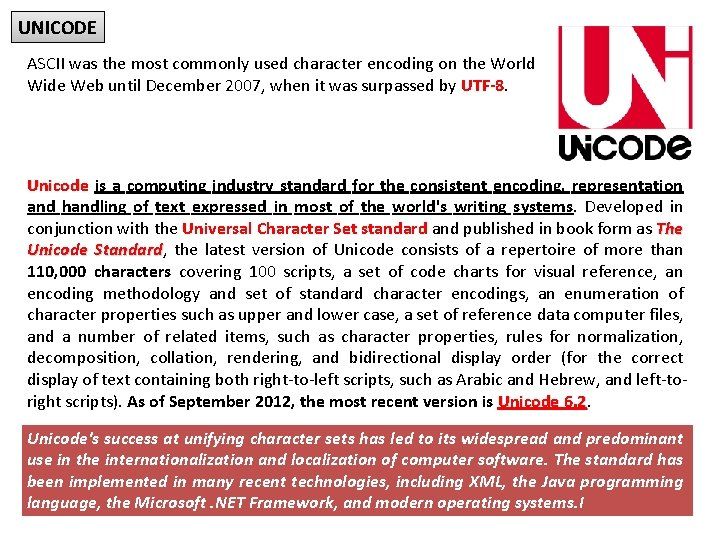UNICODE ASCII was the most commonly used character encoding on the World Wide Web until December 2007, when it was surpassed by UTF-8. Unicode is a computing industry standard for the consistent encoding, representation and handling of text expressed in most of the world's writing systems. Developed in conjunction with the Universal Character Set standard and published in book form as The Unicode Standard, Standard the latest version of Unicode consists of a repertoire of more than 110, 000 characters covering 100 scripts, a set of code charts for visual reference, an encoding methodology and set of standard character encodings, an enumeration of character properties such as upper and lower case, a set of reference data computer files, and a number of related items, such as character properties, rules for normalization, decomposition, collation, rendering, and bidirectional display order (for the correct display of text containing both right-to-left scripts, such as Arabic and Hebrew, and left-toright scripts). As of September 2012, the most recent version is Unicode 6. 2 Unicode's success at unifying character sets has led to its widespread and predominant use in the internationalization and localization of computer software. The standard has been implemented in many recent technologies, including XML, the Java programming language, the Microsoft. NET Framework, and modern operating systems. I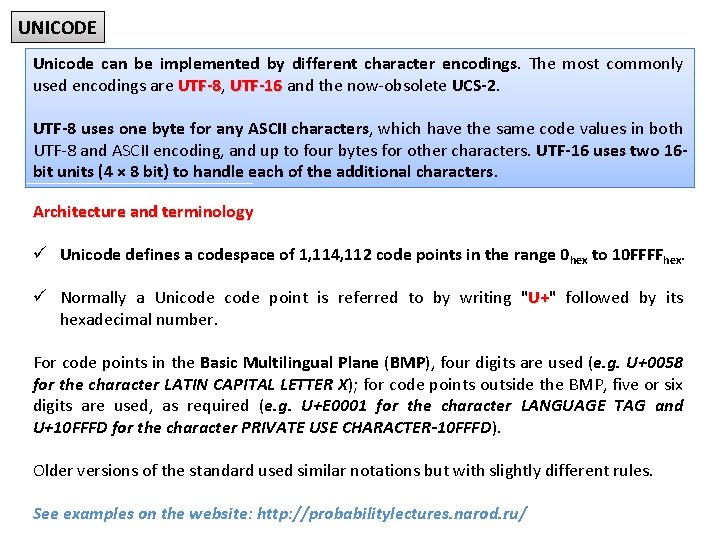UNICODE Unicode can be implemented by different character encodings. The most commonly used encodings are UTF-8, UTF-8 UTF-16 and the now-obsolete UCS-2. UTF-8 uses one byte for any ASCII characters, which have the same code values in both UTF-8 and ASCII encoding, and up to four bytes for other characters. UTF-16 uses two 16 bit units (4 × 8 bit) to handle each of the additional characters. Architecture and terminology ü Unicode defines a codespace of 1, 114, 112 code points in the range 0 hex to 10 FFFFhex. ü Normally a Unicode point is referred to by writing "U+" U+ followed by its hexadecimal number. For code points in the Basic Multilingual Plane (BMP), four digits are used (e. g. U+0058 for the character LATIN CAPITAL LETTER X); for code points outside the BMP, five or six digits are used, as required (e. g. U+E 0001 for the character LANGUAGE TAG and U+10 FFFD for the character PRIVATE USE CHARACTER-10 FFFD). Older versions of the standard used similar notations but with slightly different rules. See examples on the website: http: //probabilitylectures. narod. ru/Data Representation - Audio Sound is perceived when a series of air compressions vibrate a membrane in our ear, which sends signals to our brain. A stereo system sends an electrical signal to a speaker to produce sound. This signal is an analog representation of the sound wave. The voltage in the signal varies in direct proportion to the sound wave. To digitize the signal we periodically measure the voltage of the signal and record the appropriate numeric value. The process is called sampling. In general, a sampling rate of around 40, 000 times per second is enough to create a very good high quality sound reproduction.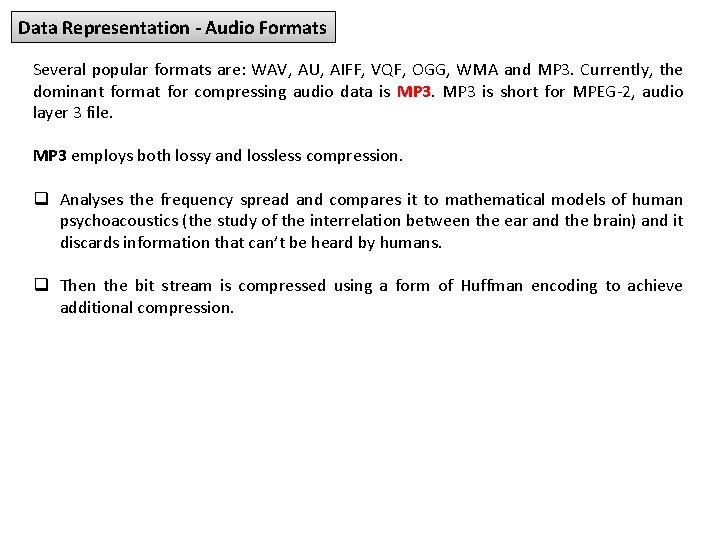Data Representation - Audio Formats Several popular formats are: WAV, AU, AIFF, VQF, OGG, WMA and MP 3. Currently, the dominant format for compressing audio data is MP 3 is short for MPEG-2, audio layer 3 file. MP 3 employs both lossy and lossless compression. q Analyses the frequency spread and compares it to mathematical models of human psychoacoustics (the study of the interrelation between the ear and the brain) and it discards information that can’t be heard by humans. q Then the bit stream is compressed using a form of Huffman encoding to achieve additional compression.Data Representation - Images and Graphics Colour is our perception of the various frequencies of light that reach the retinas of our eyes. Our retinas have three types of colour photoreceptor cone cells that respond to different sets of frequencies. These photoreceptor categories correspond to the colours of red, green, and blue. Colour is often expressed in a computer as an RGB (red-green-blue) red-green-blue value, which is actually three numbers that indicate the relative contribution of each of these three primary colours. For example, an RGB value of (255, 0) maximizes the contribution of red and green, and minimizes the contribution of blue, which results in a bright yellow.Data Representation - Images and Graphics The amount of data that is used to represent a colour is called the colour depth. q Hi. Colour is a term that indicates a 16 -bit colour depth. Five bits are used for representing the R and B components. Six bits are used for representing the G component, because the human eye is more sensitive to G; q True. Colour indicates a 24 -bit colour depth. Therefore, each number in an RGB value is represented using eight bits. Digitized Images and Graphics Digitizing a picture is the act of representing it as a collection of individual dots called pixels. The word “pixel” was derived from the words, “picture element”. The number of pixels used to represent a picture is called the resolution.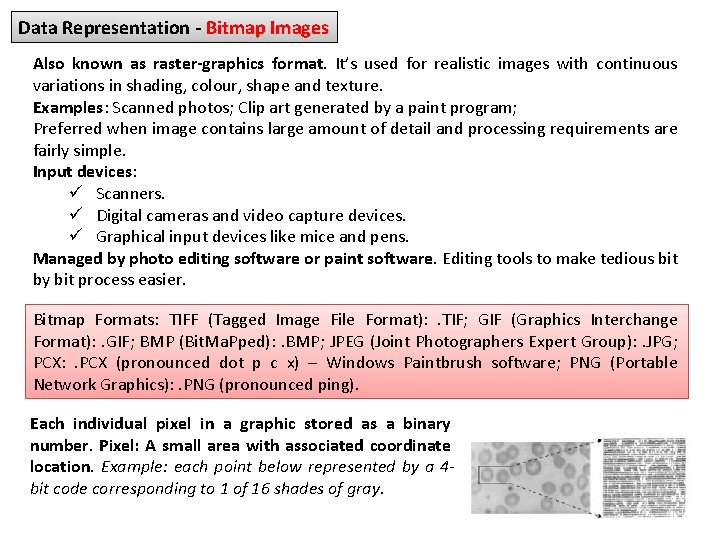Data Representation - Bitmap Images Also known as raster-graphics format. It’s used for realistic images with continuous variations in shading, colour, shape and texture. Examples: Scanned photos; Clip art generated by a paint program; Preferred when image contains large amount of detail and processing requirements are fairly simple. Input devices: ü Scanners. ü Digital cameras and video capture devices. ü Graphical input devices like mice and pens. Managed by photo editing software or paint software. Editing tools to make tedious bit by bit process easier. Bitmap Formats: TIFF (Tagged Image File Format): . TIF; GIF (Graphics Interchange Format): . GIF; BMP (Bit. Ma. Pped): . BMP; JPEG (Joint Photographers Expert Group): . JPG; PCX: . PCX (pronounced dot p c x) – Windows Paintbrush software; PNG (Portable Network Graphics): . PNG (pronounced ping). Each individual pixel in a graphic stored as a binary number. Pixel: A small area with associated coordinate location. Example: each point below represented by a 4 bit code corresponding to 1 of 16 shades of gray.Data Representation - Object Images A vector-graphics format describe an image in terms of lines and geometric shapes. A vector graphic is a series of commands that describe a line’s direction, thickness, and colour. The file size for these formats tend to be small because every pixel does not have to be accounted for. Vector graphics can be resized mathematically, and these changes can be calculated dynamically as needed. However, vector graphics is not good for representing real-world images. Created by drawing packages or output from spreadsheet data graphs. Computer translates geometric formulas to create the graphic. Storage space required depends on image complexity number of instructions to create lines, shapes, fill patterns. Cannot represent photos or paintings. For Example: Objects seen in movies like Shrek, Toy Story, Madagascar. Most object image formats are proprietary. Files extensions include. wmf, . dxf, . mgx, and. cgm. Popular Object Graphics Software: Macromedia Flash; Micrographx Designer; Corel. Draw (vector illustration, layout, bitmap creation, image-editing, painting and animation software); Autodesk Auto. CAD; W 3 C SVG (Scalable Vector Graphics) based on XML Web description language – Not proprietary.Data Representation - Bitmap vs. Object Images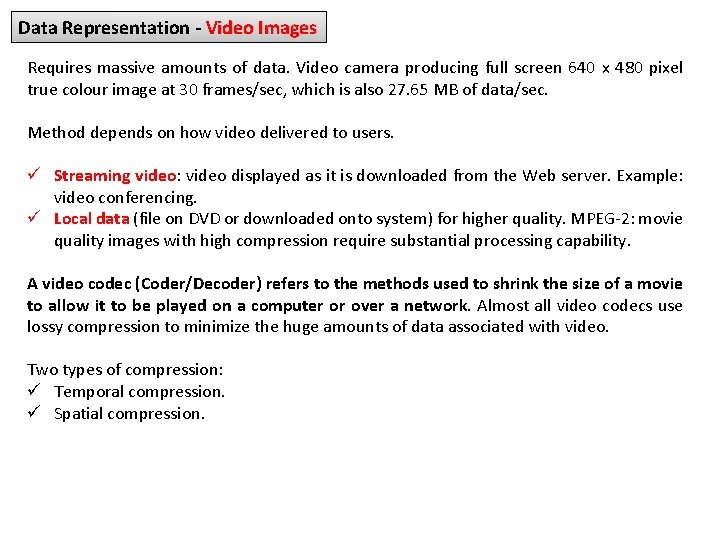Data Representation - Video Images Requires massive amounts of data. Video camera producing full screen 640 x 480 pixel true colour image at 30 frames/sec, which is also 27. 65 MB of data/sec. Method depends on how video delivered to users. ü Streaming video: video displayed as it is downloaded from the Web server. Example: video conferencing. ü Local data (file on DVD or downloaded onto system) for higher quality. MPEG-2: movie quality images with high compression require substantial processing capability. A video codec (Coder/Decoder) refers to the methods used to shrink the size of a movie to allow it to be played on a computer or over a network. Almost all video codecs use lossy compression to minimize the huge amounts of data associated with video. Two types of compression: ü Temporal compression. ü Spatial compression.Thanks for attention